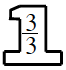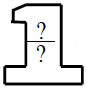### Home > CC2MN > Chapter 5 Unit 5 > Lesson CC2: 5.1.1 > Problem5-11

5-11.

Fill in the missing numbers in each number sentence.  Homework Help ✎

1. $\frac{5}{8}\cdot$$=\frac{?}{?}$

Multiply both the numerators and denominators of the fractions.

$\frac{15}{24}$

1. $\frac{9}{15}\cdot$$=\frac{?}{60}$

What is $60$ divided by $15$? Use this reasoning to find the value of the Giant One.

$\text{Giant One} = \frac{4}{4}$

1. $\frac{7}{20}\cdot$$=\frac{?}{110}$

Follow the steps in part (b).

1. $\frac{44}{100}\cdot$$=\frac{?}{60}$

Follow the steps in part (b).

$\frac{0.6}{0.6}$

1. What strategies did you use to find the numbers in the Giant One in parts (b), (c), and (d)?

Examine your method. What steps did you take?